# Dieterici equation of state

The Dieterici equation of state, proposed in 1899 (Ref. 1) is given by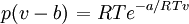$\left. p(v-b) \right.= RT e^{-a/RTv}$

where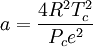$a = \frac{4R^2T_c^2}{P_ce^2}$

and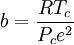$b=\frac{RT_c}{P_ce^2}$

where$p$ is the pressure,$T$ is the temperature and$R$ is the molar gas constant.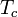$T_c$ is the critical temperature and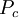$P_c$ is the pressure at the critical point.